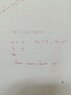# Convert to a first order ODE

• LSMOG
In summary: I don't know what other helpers think, but every now and then we get here question on a very standard problem the answer or solution method to which is the first chapter, or the first page of a chapter in any textbook on the subject. Helpers are not here to re-write the textbooks but to help out with difficulties or confusions in understanding points or difficulties students run into trying to apply the methods.In summary, this problem involves converting a second-order ODE into a system of two first-order differential equations.

#### LSMOG

Moved from a technical forum, so homework template missing
What am I doing wrong here in my attachment?

#### Attachments

•15270592973801975924065.jpg
12.6 KB · Views: 391
I'll be nice and write it out for you, but this is something that you should have done yourself instead of posting a picture:
$$2 y'' -5 y' + y = 0$$
You then set ##x_1 = y##, ##x_2 = y'## and ##x_3 = y''##.

First off, that ##x_3## shouldn't be there. You only introduce new variables up to one order less than the ODE.

Second, when you start writing the new set of ODEs, you must write everything in terms of the new variables, so there shouldn't be any ##y##'s left.

Thanks a lot, thanks again

You are not doing something wrong because you're not really doing anything!
I don't know what other helpers think, but every now and then we get here question on a very standard problem the answer or solution method to which is the first chapter, or the first page of a chapter in any textbook on the subject. Helpers are not here to re-write the textbooks but to help out with difficulties or confusions in understanding points or difficulties students run into trying to apply the methods.

I assume you can actually solve this equation? That will be a useful check and background. The question is asking you to solve it in a particular way. It Ii almost telling you the way when it says ' convert to first-order o.d.e.'. A first order (homogeneous linear with constant coefficients) ode is something of the form
(aD + B)f(y) = 0 where D stands for d/dx and you have to find a, b, f . f(y) = 0 is another first-order ode.
Doing this is called "factorisation of the operator". You will find it in plenty of books and online sources. If you consider first some elementary examples (your example is not quite the easiest) you will begin to see this as a natural thing to do, not something taken out of a hat. If you apply the "operator" (aD + b) to a first order differential equation, you will get a second order differential equation.

Last edited:
•Mark44
epenguin said:
The question is asking you to solve it in a particular way. It Ii almost telling you the way when it says ' convert to first-order o.d.e.'.
The thread title betrays some misunderstanding: "Convert to a first order ODE".
You can't convert a second-order ODE to a first-order ODE, but you can convert a second-order ODE into a system of two first-order differential equations.

epenguin said:
Helpers are not here to re-write the textbooks but to help out with difficulties or confusions in understanding points or difficulties students run into trying to apply the methods.
I agree. Your first resource should be your textbook, which almost certainly has an example of a problem like this.

Thanks and I am sorry colleagues